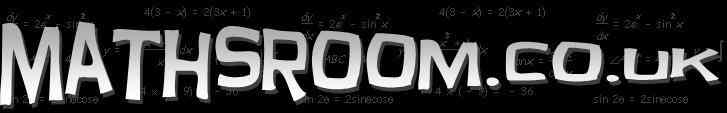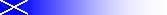Online interactive resources for the Scottish Maths curriculum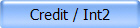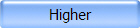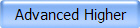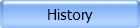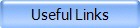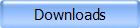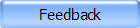General / Int1 Exam Tips Helpful tips and things to avoid in your Maths exam. Maths teaching resources Interactive activities, games, worksheets, lesson plans and more. BBC Bitesize – Standard Grade Maths I Number, Pythagoras, Trigonometry, Algebra, Areas &Volumes, Graphs Coordinates 1 Navigate safely through the minefield using coordinates - first quadrant only. Coordinates 2 Navigate safely through the minefield using coordinates in all four quadrants. 3D-shapes (Java Applet) Teaching/Demo Every 3D shape that you could possibly think of. All 433 of them!! Geometry (Java Applet) Circle, triangles, quadrilaterals, triangles, symmetry. Angle Facts (Flash) Interactive investigation of 20 angle facts using an on-screen protractor.Distance-Time 1 (Java Applet) Create real-time D-T graph by controlling the movement of a wee man. Distance-Time 2 (Java Applet) Control the speed, direction and starting position of two runners. Watch them race then analyse the Distance-Time graph. Also includes questions and points for discussion. Distance-Time 3 (Flash) Excellent Distance, Speed & Time activity in the context of a football match. Includes video footage of Man Utd vs West Ham (Nov 2000) Scientific Notation (Flash) Explore the components of scientific notation. Includes exercises on converting between forms. Understanding Payslips Detailed explanation of each section on an average payslip. Understanding Cheques Information about writing cheques. Understanding Bank Statements Guidance on reading your bank statements.Interactive Algebra Worksheet Substitute values into expressions and evaluate. Working should be done on paper and answer inputed for correction. Solving Equations Practice Solve randomly generated equations, for example: 2x = -6, 5(x + 2) = 15, 4x - 1 = -x + 9. Working should be done on paper and answer inputed for correction. y = mx + c (Java Applet) (Java Applet) Watch the line change in real time as you alter m or c. Simultaneous Equations (Java Applet) Graphs two linear equations showing the solution as the point where the lines intersect. Pythagoras Theorem (Flash) Observe the the square on the hypotenuse as you alter the other two sides. Includes interactive exercise and on-line scientific calculator. Trigonometry (Flash) Animated introduction to right-angled trigonometry. Histogram  Bar Graph  Pie Chart  Stem & Leaf Diagram (Java Applet) Interactive Statistical Diagrams View and create these using built in data or user defined data.